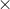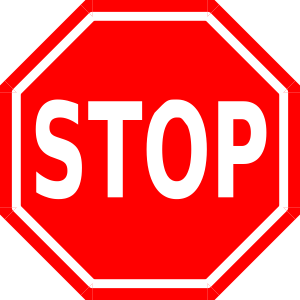5.9 Converting Metric Units

# Learning Objectives

• Use powers of ten to convert between the most commonly used metric measures.

# The Computer GameBefore leaving the science museum, Caleb found a really cool computer game all about metrics. Caleb had been practicing his metric conversions while playing at the Metric Playground, but now it was time for him to apply what he had learned.

The object of the game is to move the mountain climber up the mountain by solving problems involving metric lengths, weights and liquids. Each time a correct answer is given, the mountain climber moves up the mountain. You keep playing until the climber reaches the top.

At the beginning of the game, Caleb sees this problem on the computer screen. It is a problem that requires Caleb to use greater than or less than symbols to compare values.

5.5 grams _____ 4500 mg

Caleb is unsure of the correct answer. He decides to skip this problem by pushing the NEXT button on the computer.

Here is Caleb’s second problem.

6.7 Liters10 = _____

Caleb thought that the answer was 6700 so he entered that answer into the computer.

TRY AGAIN popped up on his screen.

Finally Caleb decided to try one more problem.

1550 kg is one hundred times lighter than _____ kilograms

Caleb is stuck again.

You can help Caleb. In this lesson you will learn all about comparing metric units of length, mass and capacity. You will also learn to convert units using powers of ten.

# Guided Learning

## Convert Metric Units of Length, Mass, and Capacity Using Powers of Ten

This section combines a couple of different skills that we have already learned. We have learned all about metrics and about how to convert metric units of length, mass, and capacity. We have also learned how to multiply decimals using powers of ten such as 10, 100, 1,000.

How can we put these two skills together?

We can put them together by converting metric units using powers of ten. This will require us to move the decimal point as we did in earlier lessons. Let’s look at an example.

### Example A

Convert 150 cm into mm by multiplying by a power of ten.

We know that there are 10 mm in 1 cm. When we go from a larger unit to a smaller unit we multiply. Therefore, we are going to multiply 150 cm by 10.

150 cm10_____ mm

We know that when we multiply by 10 we move the decimal point one place to the right. The decimal point in a whole number is after the number. So we need to add a zero placeholder to 150.

150 cm = 1,500 mm

We can do this when we convert from a smaller unit to a larger unit too. Let’s look at this one involving capacity.

### Example B

1,250 milliliters = _____ L

We know that there are 1000 milliliters in one liter. We need to divide 1250 milliliters by 1000. To do this, we will move the decimal point three places to the left. The decimal point is after the number in a whole number.

1,250 milliliters = 1.25 Liters

We can complete this with any unit of measure as long as we know the conversion equivalents and remember how to use powers of ten to move the decimal point to the left or to the right.

## Guided Practice

Here are a few for you to try.

1. 1,340 ml = _____ liters
2. 66 grams = _____ mg
3. 1,123 m = _____ kmTake a few minutes to check your work with a peer.

Solutions:

1. 1.34
2. 66,000
3. 1.123

## Compare and Order Given Metric Measurements of Length, Mass, or Capacity

In a previous lesson, we learned that we can have metric units that are equivalents of each other. For example, 100 cm is equal to 1 meter. Because of this, 500 cm is equal to 5 meters. What if we have different metric units and different quantities?

How can we compare metric units?

To compare metric units, we have to use comparisons between the numbers. Let’s look at an example.

### Example C

4.5 m _____ 500 cm

We have two different metric units here. We have centimeters and we have meters. We can compare the units by thinking about the equivalents. If there are 100 centimeters in one meter, then 500 cm is the same as five meters. Five meters is greater than 4.5 meters.

4.5 m < 500 cm

We can work this way with metric units of length, mass, and capacity.

### Example D

7.6 kg _____ 7800 g

Which is greater? To figure this out, we need to use the equivalents that we have already learned. There are 1000 grams in 1 kg. Therefore, 7800 grams becomes 7.8 kilograms.

We know from our work with decimals that 7.6 is less than 7.8. Now we can compare them.

7.6 kg < 7800 g

## Guided Practice

Take a minute to compare a few of these on your own.

1. 6.5 kg _____ 50000 g
2. 500 mL _____ .5 liters
3. 7000 m _____ 7.1 kmTake a few minutes to check your work with a peer.

Solutions:

1. 6,500 g  >  50000 g
2. 500 mL = 500 mL
3. 7 km < 7.1 km

# Real Life Example Completed

The Computer GameNow we are ready to help Caleb with his computer game. Here is the problem once again.

Before leaving the science museum, Caleb found a really cool computer game all about metrics. Caleb had been practicing his metric conversions while playing at the Metric Playground, but now it was time for him to apply what he had learned.

The object of the game is to move the mountain climber up the mountain by solving problems involving metric lengths, weights, and liquids. Each time a correct answer is given, the mountain climber moves up the mountain. You keep playing until the climber reaches the top.

At the beginning of the game, Caleb sees this problem on the computer screen. It is a problem that requires Caleb to use greater than or less than symbols to compare two values.

5.5 grams _____ 4500 mg

Caleb is unsure of the correct answer. He decides to skip this problem by pushing the NEXT button on the computer.

Here is Caleb’s second problem.

6.7 Liters x 10 = _____

Caleb thought that the answer was 6,700 so he entered that answer into the computer.

TRY AGAIN popped up on his screen.

Finally Caleb decided to try one more problem.

1550 kg is one hundred times lighter than _____ kilograms.

Caleb is stuck again.

We are going to help Caleb answer all three questions. Let’s start with the first one.

5.5 grams _____ 4500 mg

There are 1000 mg in 1 gram. Therefore, if we change the 4500 milligrams to grams by moving the decimal point three places to the left, we end up with 4.5 grams. 5.5 is greater than 4.5.

5.5 grams > 4500 mg

The second problem requires multiplying by powers of ten.

6.7 liters10_____

To multiply by a power of ten we move the decimal point to the right. Here we are multiplying by 10, so we move the decimal point one place to the right.

6.7 liters1067 liters

Our final problem involves division by powers of ten.

1550 kg is one hundred times lighter than _____ kilograms

We want to make 1550 kg 100 times lighter. To do this, we divide by 100. To divide by 100, a power of 10, we move the decimal point two places to the left.

15.5 kg is our answer.

# Review

• Capacity is the measure of volume.
• Milliliter is the smallest unit. Milliliters are most useful when measuring really tiny things.
• Centimeter is the next smallest unit, ten times bigger than a milliliter.
• Liter is this is the “base” unit of metric capacity.
• Kiloliter is the unit used to measure larger capacities, like a swimming pool.
• Mass is the measure of weight.
• Milligram is the smallest unit. Milligrams are most useful when measuring really tiny things.
• Gram is the “base” unit of metric mass.
• Kilogram is the unit used to measure larger mass, it’s about 2.5 pounds.

# Video Resources

Measuring Metric Length Video